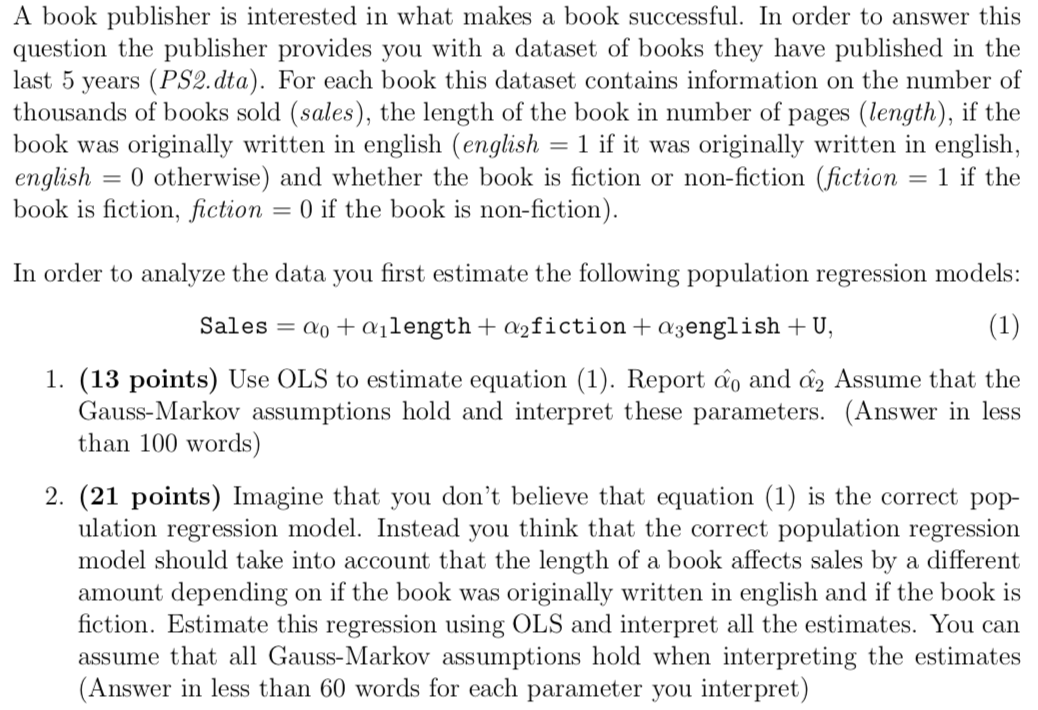### Create an Account

Home / Questions / A book publisher is interested in what makes a book successful In order to answer this que...

# A book publisher is interested in what makes a book successful In order to answer this question the publisher provides you wA book publisher is interested in what makes a book successfulA book publisher is interested in what makes a book successful. In order to answer this question the publisher provides you with a dataset of books they have published in the last 5 years (PS2.dta). For each book this dataset contains information on the number of thousands of books sold (sales), the length of the book in number of pages (length), if the book was originally written in english (english = 1 if it was originally written in english, english = 0 otherwise) and whether the book is fiction or non-fiction (fiction = 1 if the book is fiction, fiction = 0) if the book is non-fiction). In order to analyze the data you first estimate the following population regression models: Sales = Qo +ajlength+Q2fiction +azenglish + U, 1. (13 points) Use OLS to estimate equation (1). Report âo and Q2 Assume that the Gauss-Markov assumptions hold and interpret these parameters. (Answer in less than 100 words) 2. (21 points) Imagine that you don&#39;t believe that equation (1) is the correct pop- ulation regression model. Instead you think that the correct population regression model should take into account that the length of a book affects sales by a different amount depending on if the book was originally written in english and if the book is fiction. Estimate this regression using OLS and interpret all the estimates. You can assume that all Gauss-Markov assumptions hold when interpreting the estimates (Answer in less than 60 words for each parameter you interpret)

Feb 07 2020 View more View LessGet Solution